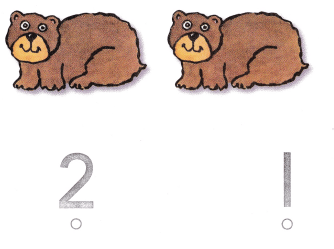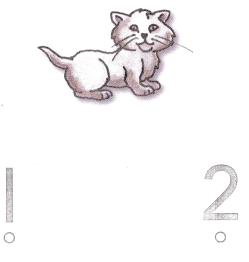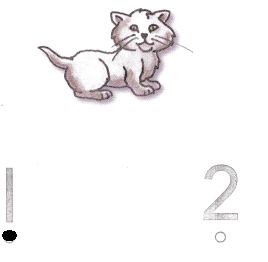# Texas Go Math Kindergarten Lesson 1.1 Answer Key Model and Count 1 and 2

Refer to our Texas Go Math Kindergarten Answer Key Pdf to score good marks in the exams. Test yourself by practicing the problems from Texas Go Math Kindergarten Lesson 1.1 Answer Key Model and Count 1 and 2.

## Texas Go Math Kindergarten Lesson 1.1 Answer Key Model and Count 1 and 2

Explore

DIRECTIONS: Place a counter on each object in the set as you IIS count them. Move the counters to the five frame. Draw the counters.Explanation:
Placed the counter showing the picture

Share and Show

DIRECTIONS: 1-2. Place a counter on each object in the set as you count them. Tell how many counters. Move the counters to the five frame. Draw the counters.
Question 1.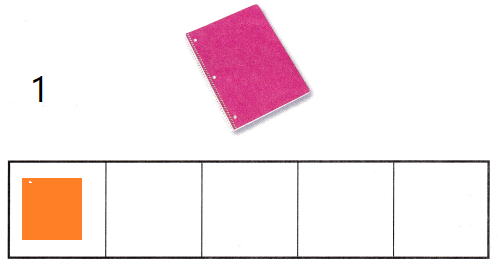Explanation:
Placed a counter on each object in the set as we count them.
Counted the number
Drawn a image to show the number

Question 2.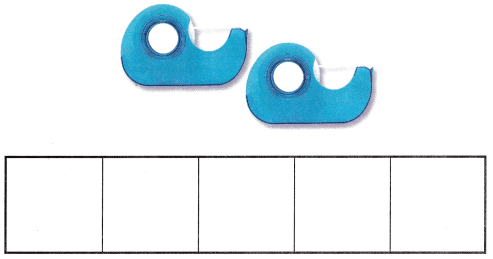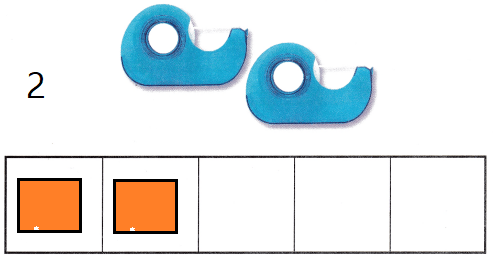Explanation:
Explanation:
Placed a counter on each object in the set as we count them.
Counted the number
Drawn a image to show the number

DIRECTIONS: 3-6. Say the number. Count out that many counters in the five frame. Draw the counters.

Question 3.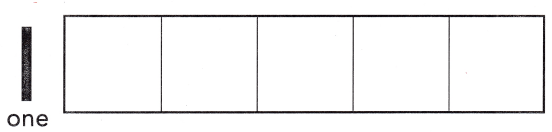Explanation:
Drawn 1 star

Question 4.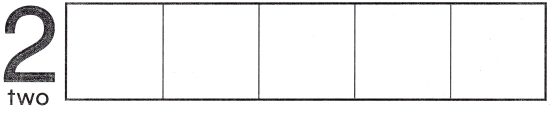Explanation:
Drawn 2 stars

Question 5.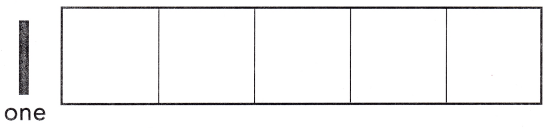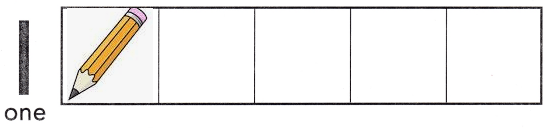Explanation:
Drawn 1 pencil

Question 6.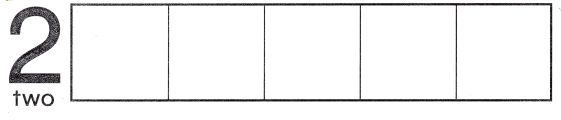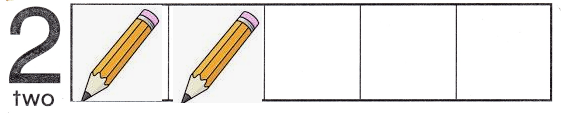Explanation:
Drawn 2 pencils.

HOME ACTIVITY • Ask your child to show a set that has one or two objects. such as books or buttons. Hove him or her point to each object as he or she counts it to find out how many objects are in the set.Explanation:
Counted and written the numbers.

Problem Solving

DIRECTIONS: 7. Count the objects in each set. Circle the set that has two objects. 8. Choose the correct answer. What number does the model show?

Question 7.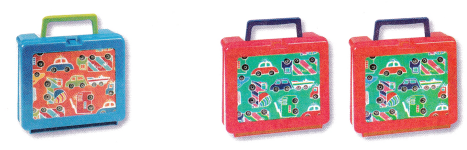Explanation:
Drawn a circle that has 2 objects.

Question 8.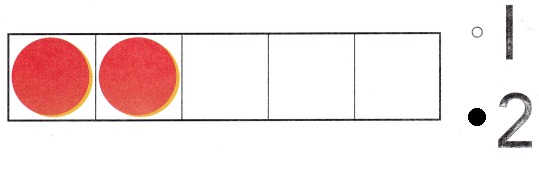Explanation:
Circled the number to the correct object count

### Texas Go Math Kindergarten  Lesson 1.1 Homework and Practice Answer Key

DIRECTIONS: 1. Count the objects in each set. Circle the set that has one object. 2. Count the objects in each set. Circle the set that has two objects. 3. Count the objects in each set. Circle the set that has one object.

Question 1.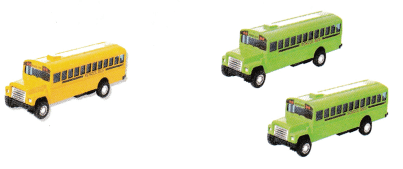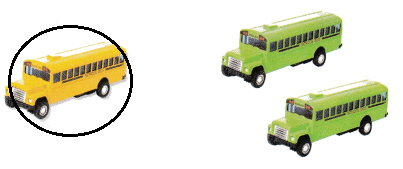Explanation:
Counted the objects in each set.
Circled the set that has one object.

Question 2.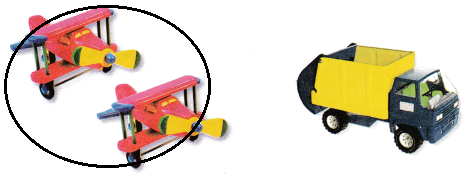Explanation:
Counted the objects in each set.
Circled the set that has two objects.

Question 3.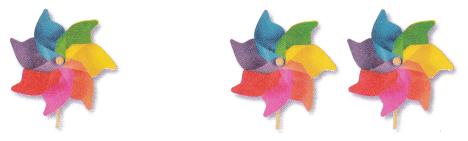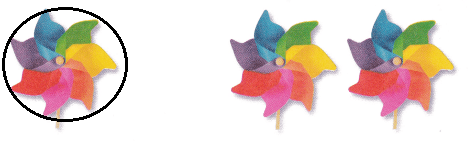Explanation:
Counted the objects in each set.
Circled the set that has two objects.

DIRECTIONS: Choose the correct answer. 4. How many bears are there? 5. How many cats are there?

Lesson Check

Question 4.